×#### Thank you for registering.

One of our academic counsellors will contact you within 1 working day.

Click to Chat

1800-1023-196

+91-120-4616500

CART 0

• 0

MY CART (5)

Use Coupon: CART20 and get 20% off on all online Study Material

ITEM
DETAILS
MRP
DISCOUNT
FINAL PRICE
Total Price: Rs.

There are no items in this cart.
Continue Shopping• Complete Physics Course - Class 11
• OFFERED PRICE: Rs. 2,968
• View Details

```Prism

Table of Content

Refraction through a Prism

Refraction through a Prism for Small Angle of incidence

Dispersion

​Reason for Dispersion

Refraction through a Prism

Solved Problems

Related Resources

Refraction through a PrismA prism is a wedge-shaped body made from a refracting medium bounded by two plane faces inclined to each other at some angle. The two plane faces are called are the refracting faces and the angle included between these two faces is called the angle of prism or the refracting angle.

In the below figure (1), ABC represents the principal section of a glass-prism having ∠A as its refracting angle.

A ray KL is incident on the face AB at the point F where N1LO is the normal and ∠i1 is the angle of incidence. Since the refraction takes place from air to glass, therefore, the refracted ray LM bends toward the normal such that ∠r1 is the angle of refraction. If µ be the refractive index of glass with respect to air, thenµ = sin i/sin r     (By Snell’s law)

The refracted ray LM is incident on the face AC at the point M where N2MO is the normal and ∠r2 is the angle of incidence. Since the refraction now takes place from denser to rarer medium, therefore, the emergent ray MN such that ∠i2 is the angle of emergence.

In the absence of the prism, the incident ray KL would have proceeded straight, but due to refraction through the prism, it changes its path along the direction PMN. Thus, ∠QPN gives the angle of deviation ‘δ’, i.e., the angle through which the incident ray gets deviated in passing through the prism.

Thus,   δ = i1 – r1 + i2 -r2     ….... (1)

δ = i1 + i2 – (r1 + r2 )

∠ALO + ∠AMO = 2rt∠s                [Since, ∠ALO = ∠AMO = 90º]

So, ∠LAM +∠LOM = 2rt∠s           [Since, Sum of four ∠s of a quadrilateral = 4 rt∠s]    ….... (2)

Also in ?LOM,

∠r1 +∠r2 + ∠LOM = 2rt∠s         …... (3)

Comparing (2) and (3), we get

∠LAM = ∠r1 +∠r2

A = ∠r1 +∠r2

Using this value of ∠A, equation (1) becomes,

δ = i1 + i2 - A

or i1 + i2 = A + δ                   …... (4)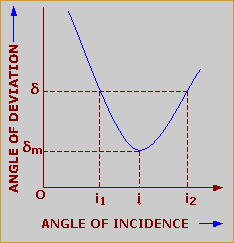The angle of deviation of a ray of light in passing through a prism not only depends upon its material but also upon the angle of incidence. The above figure (2) shows the nature of variation of the angle of deviation with the angle of incidence. It is clear that an angle of deviation has the minimum value ‘δm’ for only one value of the angle of incidence. The minimum value of the angle of deviation when a ray of light passes through a prism is called the angle of minimum deviation.

The figure (3) shows the prism ABC, placed in the minimum deviation position. If a plane mirror M is placed normally in the path of the emergent ray MN the ray will retrace its original path in the opposite direction NMLK so as to suffer the same minimum deviation dm.

In the minimum deviation position, ∠i1 = ∠i2

and so ∠r1 = ∠r2 = ∠r    (say)

Obviously, ∠ALM = ∠LMA = 90º – ∠r

Thus, AL = LM

and so LM l l BC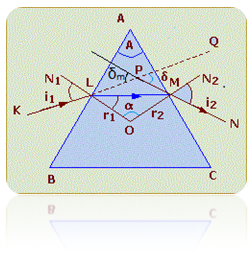Hence, the ray which suffers minimum deviation possess symmetrically through the prism and is parallel to the base BC.

Since for a prism,

∠A = ∠r1 + ∠r2

So, A = 2r      (Since, for the prism in minimum deviation position, ∠r1 = ∠r2 = ∠r)

or r = A/2        …...(5)

Again, i1 + i2 = A + δ

or i1 + i1 = A + δm         (Since, for the prism in minimum deviation position, i1 = i2 and δ = δm)

2i1 = A + δm

or i1 = (A + δm) / 2           …... (6)

Now  µ = sin i1/sin r1 = sin i1/sin r

µ = sin [(A + δm) / 2] / sin (A/2)               …... (7)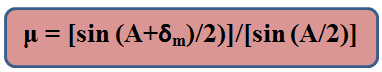Equation (7) gives the relation between the refractive index of the material of the prism and the minimum deviation.

Grazing Incidence:-

When i = 90°, the incident ray grazes along the surface of the prism and the angle of refraction inside the prism becomes equal to the critical angle for glass - air. This is known as grazing incidence.

Grazing Emergence:-

When e = 90°, the emergent ray grazes along the prism surface. This happens when the light ray strikes the second face of the prism at the critical angle for glass - air. This is known as grazing emergence.

Maximum Deviation:-

The angle of deviation is same for both the above cases (grazing incidence & grazing emergence) and it is also the maximum possible deviation if the light ray is to emerge out from the other face without any total internal reflection.When a ray of light suffers minimum deviation through a prism.

Angle of incidence is equal to the angle of emergence.

Ray of light passing through the prism is parallel to the base of the prism.

Angle of refraction inside the material of prism is equal to half the angle of prism.

Refraction through a Prism for Small Angle of incidenceFigure (4) shows a prism having a very small refracting ∠A. a ray KL is incident on the face AB such that the angle of incidence ‘i’ is very small. Accordingly, the angle of refraction ‘r’ will also be very small. Also angles ‘r2’ and ‘i2’ must also be very small. If ‘µ’ be the refractive index of the material of the prism, then

µ = sin i1 / sin r1

µ = i1 / r1

(Since sin i1 = i1 and sin r1 = r1 when angles i and r1 are very small)

or i1 = µr1

Similarly,i2 = µr2

Thus,  i1 + i2 =  µr1 +  µr2 = µ (r1 +  r2)

Or, i1 + i2 = µA      (Since, A = r1 +  r2)          …....(8)

Also, i1 + i2 = A + δ                   …... (9)

Therefore, from (8) and (9), we get,

A + δ =  µA

Or, δ = µA – A

Or, δ = A(µ – 1)This relation shows that the angle of deviation ‘δ’ is independent of the angle of incidence, provided it is small. In other words, if ‘i1’ changes, ‘i2’ will also change accordingly.

Dispersion

With the help of Newton’s disc experiment, it can be proved that white light is composed of the seven colours. Newton’s disc is a disc divided into seven parts. Different parts are coated with pure pigment colours in the order: violet, indigo, blue, green, yellow, orange and red. On rotating the disc at a high speed, all the colours merge into one another due to persistence of vision, giving a resultant white impression.

When a ray of white light passes through a prism, it splits up into its constituent’s colours, producing a band of colours on the screen XY as shown in the figure.

This splitting up of light into its constituent colours is called dispersion.

Reason for Dispersion

Refractive index of a transparent medium depends upon the nature of light (i.e., its wave-length) passing through it. According to Cauchy’s formula, refractive index of a material is given by,

µ = A + B/λ2 +.......                 (10)Here, ‘A’ and ‘B’ are constants and ‘λ’ is the wave-length of light. Medium has greater refractive index for light and smaller wave-length. Since violet light has smaller wave-length than that for red light, i.e.,λv < λr.

Thus,  µv > µr

Where ‘µv’ and ‘µr’ are the refractive indices of the medium for violet and red light respectively. In case of a prism,

µ = sin [(A + δm) / 2] / sin (A/2)

Since ‘A’; the angle of prism, is same for all the colours and ‘µv’ and ‘‘µr’ are different, above equation can only be satisfied if all the colours have different values of ‘dm’, i.e., they come out along different paths and hence, the phenomenon of dispersion.

Watch this Video for more reference

Refraction through a Prism

As a ray of light is incident on one of the refracting faces of a prism and proceeds through the prism, it undergoes following two changes:

(a) Deviation      (b) Dispersion

(a) Deviation:- A ray of monochromatic light (light possessing one wave-length only), while passing through a prism suffers a change in its path, the phenomenon is known as deviation.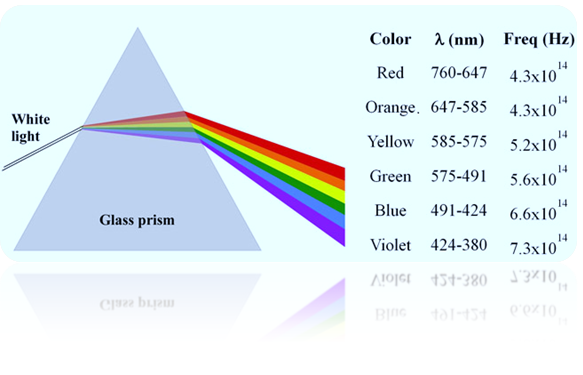it can be shown that the deviation ‘d’ suffered by a ray, provided it is incident on one of its faces at a very small angle, is given by

δ = ( µ – 1) A              ….... (11)

where ‘A’ is the refracting angle of the prism and ‘µ’ is the refractive index of the material of prism for that particular wave-length of light.

(b) Dispersion:- A ray of light (containing more than one wave-lengths), while passing through the prism splits up into a number of rays. This phenomenon is called dispersion.From equation (10) it is clear that deviation suffered by a ray depends upon the refractive index ‘µ’ of the material of prism for that wavelength. So, different wave-lengths contained in the incident ray suffer different deviations. hence, these constituent rays will emerge along different paths.

Consider a ray of white light incident on one of the faces AB of prism ABC. After passing through the prism, it splits up into its constituent colours with violet and red as its extreme colours.

Let ‘µv’ and ‘µr’ be the refractive indices of the material of prism for violet and red colours; then the corresponding deviations are given by,

δv = ( µv – 1) A

δr = ( µr – 1) A

Since, µv > µr

Therefore, ‘δv’ is greater than ‘δr’.

Other colours possess deviation in between ‘δv’ and ‘δr’. Therefore, the colours, in the dispersed beam, will be spread with in a cone of angle ‘δv – δr’.

‘δv – δr’ is called angular dispersion produced by the prism and is given by

δv – δr = [(µv – 1)A] – [(µr – 1) A]

Or, δv – δr = (µv – µr) A                               …... (12)

Dividing equation (12) by (11), we get

δv – δr /δ  = (µv – µr) A  / (µ – 1) A

δv – δr / δ = (µv – µr) / (µ – 1) = ω

Where ‘ω’ is called the dispersive power of the prism.

Dispersive power of a prism is defined as the ratio between angular dispersion to mean deviation produced by the prism.

If dµ denotes the difference between the refractive indices of material of prism for violet and red light,

ω = δµ / µ – 1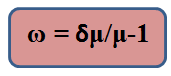Here ‘µ’ is the refractive index of prism for a mean colour. A mean colour is that colour whose wavelength lies in between that of violet and red. For white light, yellow colour is, generally, taken to be the mean colour.

Since µv is always greater than µr, the dispersive power of a prism is always positive. It depends upon the type of glass used. It is different for crown glass and for flint glass.

Solved Problems

Problem 1:

A ray of light passing through a glass prism of refracting angle 60º, undergoes a minimum deviation of 30º. Calculate the velocity of light in glass if the velocity of light in air is 3×1010 cm s-1.

Solution:-

Here, A = 60º,δm = 30º, c = 3×1010 cm s-1

Now,  µ = sin [(A + δm) / 2] / sin (A/2)               …... (i)

Also refractive index of a medium is given as

µ = velocity of light on air /velocity of light in medium = c/v                  …... (ii)

From (i) and (ii), we get,

c/v = sin [(A + δm) / 2] / sin (A/2)

or 3×1010 / v = sin [(60º + 30º) / 2] / sin (60º/2)

= sin 45° /sin 30° = (1/√2)×2 =√2

Therefore, v =  3×1010 /√2 = (3×1010) / 1.414 = 2.12×1010 cm s-1

From the above observation we conclude that, the velocity of light in glass would be

2.12×1010 cm s-1.

Problem 2:-

The refractive index of the material of a prism is 1.5. When the prism is placed in minimum deviation position, the angle of incidence is 51º. Calculate the angle of prism and the angle of minimum deviation.

Solution:-

Here    µ = 1.5, i = 51º,  A = ?,  δm = ?

Now  i1 + i2 = A + δ

Or   2i1 = A +  δm    (Since, i1= i2, for a prism in minimum deviation position)

102º =  A +  δm

Again, µ = sin [(A + δm) / 2] / sin (A/2)

1.5 = sin [102º/ 2] / sin (A/2)

= sin 51º / sin (A/2) = 0.7771/ sin (A/2)

So, sin (A/2) = 0.7771/1.5 = 0.5180

Or, A/2 = 31º 12?

Or, A = 62º 24?

Thus, dm = 102º – 62° 24? = 39° 36?

From the above observation we conclude that, the angle of prism would be 62º 24? and the angle of minimum deviation would be 39° 36?.Question 1:

In a prism which of the following phenomenon takes place.

(a) total internal reflection

(b) refraction

(c) deviation

(d) dispersion

(e) All the above

Question 2:

White light is incident on an equilateral prism. In the position of minimum deviation.

(a) angle of incidence is equal to angle of emergence

(b)the ray inside the prism is parallel to its base

(c) the angle of refraction inside the prism is equal to the angle of prism.

(d) the dispersion of all colours is same.

Question 3:

14. A ray of white light, incident upon a glass prism, is dispersed into its various color components. Which one of the following colors experiences the greatest amount of refraction?

(a) orange (b) violet  (c) red  (d) green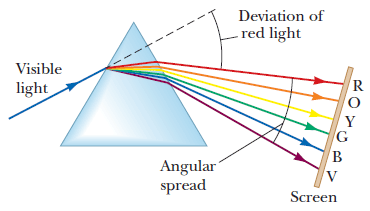Question 4:

In the spectrum of white light which colour is deviated maximum

(a) red  (b) yellow  (c) violet  (d) blue

Question 5:

In spectrum obtained with prism which colour is deviated maximum?

(a) violet   (b) red   (c) green   (d) orangeQ.1
Q.2
Q.3
Q.4
Q.5

e

a,b

b

a

a

Related Resources

You might like to magnification and thin lens.

For getting an idea of the type of questions asked, refer the  Previous Year Question Papers.

To read more, Buy study materials of Ray Optics and Optical Instruments comprising study notes, revision notes, video lectures, previous year solved questions etc. Also browse for more study materials on Physics here.
```### Course Features

• 101 Video Lectures
• Revision Notes
• Previous Year Papers
• Mind Map
• Study Planner
• NCERT Solutions
• Discussion Forum
• Test paper with Video Solution